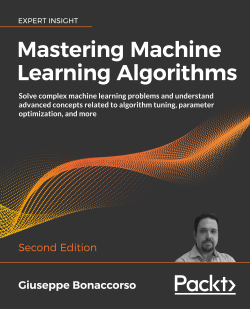# Mastering Machine Learning Algorithms - Second Edition5 (4 reviews total)
By Giuseppe Bonaccorso
• FREE Subscription Access now
• \$44.99 Print
• \$37.99 eBook
What do you get with a Packt Subscription?

• Constantly updated with 100+ new titles each month
• Breadth and depth in over 1,000+ technologies

Mastering Machine Learning Algorithms, Second Edition helps you harness the real power of machine learning algorithms in order to implement smarter ways of meeting today's overwhelming data needs. This newly updated and revised guide will help you master algorithms used widely in semi-supervised learning, reinforcement learning, supervised learning, and unsupervised learning domains.

You will use all the modern libraries from the Python ecosystem – including NumPy and Keras – to extract features from varied complexities of data. Ranging from Bayesian models to the Markov chain Monte Carlo algorithm to Hidden Markov models, this machine learning book teaches you how to extract features from your dataset, perform complex dimensionality reduction, and train supervised and semi-supervised models by making use of Python-based libraries such as scikit-learn. You will also discover practical applications for complex techniques such as maximum likelihood estimation, Hebbian learning, and ensemble learning, and how to use TensorFlow 2.x to train effective deep neural networks.

By the end of this book, you will be ready to implement and solve end-to-end machine learning problems and use case scenarios.

Publication date:
January 2020
Publisher
Packt
Pages
798
ISBN
9781838820299

# Machine Learning Model Fundamentals

Machine learning models are mathematical tools that allow us to uncover synthetic representations of external events, with the purpose of gaining better understanding and predicting future behavior. Sometimes these models have only been defined from a theoretical viewpoint, but advances in research now allow us to apply machine learning concepts to better understand the behavior of complex systems such as deep neural networks. In this chapter, we're going to introduce and discuss some fundamental elements. Skilled readers may already know these elements, but here we offer several possible interpretations and applications.

In particular, in this chapter, we're discussing the main elements of:

• Defining models and data
• Understanding the structure and properties of good datasets
• Scaling datasets, including scalar and robust scaling
• Normalization and whitening
• Selecting training, validation and test sets, including cross-validation
• The features of a machine learning model
• Learnability
• Capacity, including Vapnik-Chervonenkis capacity
• Bias, including underfitting
• Variance, including overfitting and the Cramér-Rao bound

# Models and data

Machine learning models work with data. They create associations, find out relationships, discover patterns, generate new samples, and more, working with well-defined datasets, which are homogenous collections of data points (for example, observations, images, or measures) related to a specific scenario (for example, the temperature of a room sampled every 5 minutes, or the weights of a population of individuals)

Unfortunately, sometimes the assumptions or conditions imposed on machine learning models are not clear, and a lengthy training process can result in a complete validation failure. We can think of a model as a gray box (some transparency is guaranteed by the simplicity of many common algorithms), where a vectoral input X extracted from a dataset is transformed into a vectoral output Y:

Schema of a generic model parameterized with the vector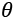and its relationship with the real world

In the preceding diagram, the model has been represented by a function that depends on a set of parameters defined by the vector. The dataset is represented by data extracted from a real-world scenario, and the outcomes provided by the model must reflect the nature of the actual relationships. These conditions are very strong in logic and probabilistic contexts, where the inferred conditions must reflect natural ones.

For our purposes, it's necessary to define models that:

• Mimic animal cognitive functions
• Learn to produce outcomes that are compatible with the environment, given a proper training set
• Learn to overcome the boundaries of the training set, by outputting the correct (or the most likely) outcome when new samples are presented

The first point is a crucial element in the AI debate. As pointed out by Darwiche (in Darwiche A., Human-Level Intelligence or Animal-Like Abilities?, Communications of the ACM, Vol. 61, 10/2018), the success of modern machine learning is mainly due to the ability of deep neural networks to reproduce specific cognitive functions (for example, vision or speech recognition). It's obvious that the outcomes of such models must be based on real-world data and, moreover, that they must possess all the features of the outcomes generated by the animals whose cognitive functions we are trying to reproduce.

We're going to analyze these properties in detail. It's important to remember that they're not simple requirements, but rather the pillars that guarantee the success or the failure of an AI application in a production environment (that is, outside of the golden world of limited and well-defined datasets).

In this section, we're only considering parametric models, although there's a family of algorithms that are called non-parametric because they're only based on the structure of the data; we're going to discuss some of them in upcoming chapters.

The task of a parametric learning process is to find the best parameter set that maximizes a target function, the value of which is proportional to the accuracy of the model, given specific input X and output Y datasets (or proportional to the error, if we're trying to minimize the error). This definition isn't very rigorous, and we'll improve it in the following sections; however, it's useful as a way to introduce the structure and the properties of the data we're using, in the context of machine learning.

## Structure and properties of the datasets

The first question to ask is: What are the natures of X and Y? A machine learning problem is focused on learning abstract relationships that allow a consistent generalization when new samples are provided. More specifically, we can define a stochastic data generating process with an associated joint probability distribution:

The process pdata represents the broadest and most abstract expression of the problem. For example, a classifier that must distinguish between male and female portraits will be based on a data generating process that theoretically defines the probabilities of all possible faces, with respect to the binary attribute male/female. It's clear that we can never work directly with pdata; it's only possible to find a well-defined formula describing pdata in a few limited cases (for example, the distribution of all images belonging to a dataset).

Even so, it's important for the reader to consider the existence of such a process, even when the complexity is too high to allow any direct mathematical modeling. A machine learning model must consider this kind of abstraction as a reference.

### Limited Sample Populations

In many cases, we cannot derive a precise distribution and we're forced to work with a limited population of actual samples. For example, a pharmaceutical experiment is aimed to understand the effectiveness of a drug on human beings. Obviously, we cannot test the drug on every single individual, nor we can imagine including all dead and future people. Nevertheless, the limited sample population must be selected carefully, in order to represent the underlying data generating process. That is, all possible groups, subgroups, and reactions must be considered.

Since this is generally impossible, it's necessary to sample from a large population. Sampling, even in the optimal case, is associated with a loss of information (unless we remove only redundancies), and therefore when creating a dataset, we always generate a bias. This bias can range from a small, negligible effect to a widespread condition that mischaracterizes the relations present in the larger population and dramatically affects the performance of a model. For this reason, data scientists must pay close attention to how a model is tested, to be sure that new samples are generated by the same process as the training samples were. If there are strong discrepancies, data scientists should warn end users about the differences in the samples.

Since we can assume that similar individuals will behave in a similar way, if the numerosity of the sample set is large enough, we are statistically authorized to draw conclusions that we can extend to the larger, unsampled part of the population. Animals are extremely capable at identifying critical features from a family of samples, and generalizing them to interpret new experiences (for example, a baby learns to distinguish a teddy-bear from a person after only seeing their parents and a few other people). The challenging goal of machine learning is to find the optimal strategies to train models using a limited amount of information, to find all the necessary abstractions that justify their logical processes.

Of course, when we consider our sample populations, we always need to assume that they're drawn from the original data-generating distribution. This isn't a purely theoretical assumption – as we're going to see, if our sample data elements are drawn from a different distribution, the accuracy of our model can dramatically decrease.

For example, if we trained a portrait classifier using 10-megapixel images, and then we used it in an old smartphone with a 1-megapixel camera, we could easily start to find discrepancies in the accuracy of our predictions.

This isn't surprising; many details aren't captured by low-resolution images. You could get a similar outcome by feeding the model with very noisy data sources, whose information content could only be partially recovered.

N values are independent and identically distributed (i.i.d.) if they are sampled from the same distribution, and two different sampling steps yield statistically independent values (that is, p(a, b) = p(a)p(b)). If we sample N i.i.d. values from pdata, we can create a finite dataset X made up of k-dimensional real vectors:

In a supervised scenario, we also need the corresponding labels (with t output values):

When the output has more than two classes, there are different possible strategies to manage the problem. In classical machine learning, one of the most common approaches is One-vs-All, which is based on training N different binary classifiers, where each label is evaluated against all the remaining ones. In this way, N-1 classifications are performed to determine the right class. With shallow and deep neural models, instead, it's preferable to use a softmax function to represent the output probability distribution for all classes:

This kind of output, where zi represents the intermediate values and the sum of the terms is normalized to 1, can be easily managed using the cross-entropy cost function, which we'll discuss in Chapter 2, Loss functions and Regularization. A sharp-eyed reader might notice that calculating the softmax output of a population allows one to obtain an approximation of the data generating process.

This is brilliant, because once the model has been successfully trained and validated with a positive result, it's reasonable to assume that the output corresponding to never-seen samples reflects the real-world joint probability distribution. That means the model has developed an internal representation of the relevant abstractions with a minimum error; which is the final goal of the whole machine learning process.

Before moving on to the discussion of some fundamental preprocessing techniques, it's worth mentioning the problem of domain adaptation, which is one of the most challenging and powerful techniques currently under development.

As discussed, animals can perform abstractions and extend the concepts learned in a particular context to similar, novel contexts. This ability is not only important but also necessary. In many cases, a new learning process could take too long, exposing the animal to all sorts of risks.

Unfortunately, many machine learning models lack this property. They can easily learn to generalize, but always under the condition of coping with samples originating from the same data generating process. Let's suppose that a model M has been optimized to correctly classify the elements drawn from p1(x, y) and the final accuracy is large enough to employ the model in a production environment. After a few tests, a data scientist discovers that p2(x, y) = f(p1(x, y)) is another data generating process that has strong analogies with p1(x, y). Its samples meet the requirements needed to be considered a member of the same global class. For example, p1(x, y) could represent family cars, while p2(x, y) could be a process modeling a set of trucks.

In this case, it's easy to understand that a transformation f(z) is virtually responsible for increasing the size of the vehicles, their relative proportions, the number of wheels, and so on. At this point, can our model M also correctly classify the samples drawn from p2(x, y) by exploiting the analogies? In general, the answer is negative. The observed accuracy decays, reaching the limit of a purely random guess.

The reasons behind this problem are strictly related to the mathematical nature of the models and won't be discussed in this book (the reader who is interested can check the rigorous paper Crammer K., Kearns M., Wortman J., Learning from Multiple Sources, Journal of Machine Learning Research, 9/2008). However, it is helpful to consider such a scenario. The goal of domain adaptation is to find the optimal methods to let a model shift from M to M' and vice versa, in order to maximize its ability to work with a specific data generating process.

It's within the limits of reasonable change, for example, for a component of the model to recognize the similarities between a car and truck (for example, they both have a windshield and a radiator) and force some parameters to shift from their initial configuration, whose targets are cars, to a new configuration based on trucks. This family of methods is clearly more suitable to represent cognitive processes. Moreover, it has the enormous advantage of allowing reuse of the same models for different purposes without the need to re-train them from scratch, which is currently often a necessary condition to achieve acceptable performances.

This topic is still enormously complex; certainly, it's too detailed for a complete discussion in this book. Therefore, unless we explicitly declare otherwise, in this book you can always assume we are working with a single data generating process, from which all the samples will be drawn.

Now, let's introduce some important data preprocessing concepts that will be helpful in many practical contexts.

### Scaling datasets

Many algorithms (such as logistic regression, Support Vector Machines (SVMs) and neural networks) show better performances when the dataset has a feature-wise null mean. Therefore, one of the most important preprocessing steps is so-called zero-centering, which consists of subtracting the feature-wise mean Ex[X] from all samples:

This operation, if necessary, is normally reversible, and doesn't alter relationships either among samples or among components of the same sample. In deep learning scenarios, a zero-centered dataset allows us to exploit the symmetry of some activation functions, driving our model to a faster convergence (we're going to discuss these details in the next chapters).

Zero-centering is not always enough to guarantee that all algorithms will behave correctly. Different features can have very different standard deviations, and therefore, an optimization that works considering the norm of the parameter vector (see the section about regularization) will tend to treat all the features in the same way. This equal treatment can produce completely different final effects; features with a smaller variance will be affected more than features with a larger variance.

In a similar way, when single features contribute to finding the optimal parameters, features with a larger variance can take control over the other features, forcing them in the context of the problem to become similar to constant values. In this way, those less-varied features lose the ability to influence the end solution (for example, this problem is a common limiting factor when it comes to regressions and neural networks). For this reason, If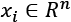,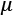, and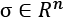and are computed considering every single feature for the whole dataset, it's often helpful to divide the zero-centered samples by the feature-wise standard deviation, obtaining the so-called z-score:

The result is a transformed dataset where most of the internal relationships are kept, but all the features have a null mean and unit variance. The whole transformation is completely reversible when it's necessary to remap the vectors onto the original space.

We can now analyze other approaches to scaling that we might choose for specific tasks (for example, datasets with outliers).

#### Range scaling

Another approach to scaling is to set the range where all features should lie. For example, if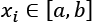so that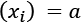and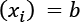, the transformation will force all the values to lie in a new range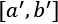, as shown in the following figure:

Schematic representation of a range scaling

Range scaling behaves in a similar way to standard scaling, but in this case, both the new mean and the new standard deviation are determined by the chosen interval. In particular, if the original features have symmetrical distributions, the new standard deviations will be very similar, even if not exactly equal. For this reason, this method can often be chosen as an alternative to a standard scaling (for example, when it's helpful to bound all the features in the range [0, 1]).

#### Robust scaling

The previous two methods have a common drawback: they are very sensitive to outliers. In fact, when the dataset contains outliers, their presence will affect the computation of both mean and standard deviation, shifting the values towards the outliers. An alternative, robust approach is based on the usage of quantiles. Given a distribution p over a range [a, b], the most common quantile, called median, 50th percentile or second quartile (Q2), is the value the splits the range [a, b] into two subsets so that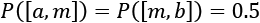. That is to say, in a finite population, the median is the value in the central position.

For example, considering the set A = {1, 2, 3, 5, 7, 9}, we have:

If we add the value 10, the set A, we get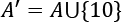:

In a similar way, we can define other percentiles or quantiles. A common choice for scaling the data is the Interquartile Range (IQR), sometimes called H-spread, defined as:

In the previous formula, Q1 is the cut-point the divides the range [a, b] so that 25% of the values are in the subset [a, Q1], while Q2 divides the range so that 75% of the values are in the subset [a, Q2]. Considering the previous set A', we get:

Given these definitions, it's easy to understand that IQR has a low sensitivity to outliers. In fact, let's suppose that a feature lies in the range [-1, 1] without outliers. In a larger dataset, we observe the interval [-2, 3]. If the effect is due to the presence of outliers (for example, the new value 10 added to A), their numerosity is much smaller than the one of normal points, otherwise they are part of the actual distribution. Therefore, we can cut them out from the computation by setting an appropriate quantile. For example, we might want to exclude from our calculations all those features whose probability is lower than 10%. In that case, we would need to consider the 5th and the 95th percentiles in a double-tailed distribution and use their difference QR = 95th – 5th.

Considering the set A', we get IQR = 5.5, while the standard deviation is 3.24. This implies that a standard scaling will compact the values less than a robust scaling. This effect becomes larger and larger as we increase the quantile range (for example, using the 95th and 5th percentiles,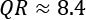). However, it's important to remember that this technique is not an outlier filtering method. All the existing values, including the outliers, will be scaled. The only difference is that the outliers are excluded from the calculation of the parameters, and so their influence is reduced, or completely removed.

The robust scaling procedure is very similar to the standard one, and the transformed values are obtained using the feature-wise formula:

Where m is the median and QR is the quantile range (for example, IQR).

Before we discuss other techniques, let's compare these methods using a dataset containing 200 points sampled from a multivariate Gaussian distribution with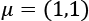and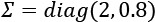:

``````import numpy as np
nb_samples = 200
mu = [1.0, 1.0]
covm = [[2.0, 0.0], [0.0, 0.8]]
X = np.random.multivariate_normal(mean=mu, cov=covm, size=nb_samples)
``````

At this point, we employ the following scikit-learn classes:

• `StandardScaler`, whose main parameters are `with_mean` and `with_std`, both Booleans, indicating whether the algorithm should zero-center and whether it should divide by the standard deviations. The default values are both `True`.
• `MinMaxScaler`, whose main parameter is `feature_range`, which requires a tuple or list of two elements (a, b) so that a < b. The default value is (0, 1).
• `RobustScaler`, which is mainly based on the parameter `quantile_range`. The default is (25, 75) corresponding to the IQR. In a similar way to `StandardScaler`, the class accepts the parameters `with_centering` and `with_scaling`, that selectively activate/deactivate each of the two functions.

In our case, we're using the default configuration for `StandardScaler`, `feature_range=(-1, 1)` for `MinMaxScaler`, and `quantile_range=(10, 90)` for `RobustScaler`:

``````from sklearn.preprocessing import StandardScaler, RobustScaler, MinMaxScaler
ss = StandardScaler()
X_ss = ss.fit_transform(X)
rs = RobustScaler(quantile_range=(10, 90))
X_rs = rs.fit_transform(X)
mms = MinMaxScaler(feature_range=(-1, 1))
X_mms = mms.fit_transform(X)
``````

The results are shown in the following figure:

Original dataset (top left), range scaling (top right), standard scaling (bottom left), and robust scaling (bottom right)

In order to analyze the differences, I've kept the same scale for all the diagrams. As it's possible to see, the standard scaling performs a shift of the mean and adjusts the points so that it's possible to consider them as drawn from N(0, I). Range scaling behaves in almost the same way and in both cases, it's easy to see how the variances are negatively affected by the presence of a few outliers.

In particular, looking at the result of range scaling, the shape is similar to an ellipse and the roundness—implied by a symmetrical distribution—is obtained by including also the outliers. Conversely, robust scaling is able to produce an almost perfect normal distribution N(0, I) because the outliers are kept out of the calculations and only the central points contribute to the scaling factor.

We can conclude this section with a general rule of thumb: standard scaling is normally the first choice. Range scaling can be chosen as a valid alternative when it's necessary to project the values onto a specific range, or when it's helpful to create sparsity. If the analysis of the dataset has highlighted the presence of outliers and the task is very sensitive to the effect of different variances, robust scaling is the best choice.

#### Normalization

One particular preprocessing method is called normalization (not to be confused with statistical normalization, which is a more complex and generic approach) and consists of transforming each vector into a corresponding one with a unit norm given a predefined norm (for example, L2):

Given a zero-centered dataset X, containing points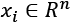, the normalization using the L2 (or Euclidean) norm transforms each value into a point lying on the surface of a hypersphere with unit radius, and centered in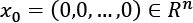(by definition all the points on the surface have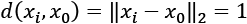).

Contrary to the other methods, normalizing a dataset leads to a projection where the existing relationships are kept only in terms of angular distance. To understand this concept, let's perform a normalization of the dataset defined in the previous example, using the scikit-learn class `Normalizer` with the parameter `norm='l2'`:

``````from sklearn.preprocessing import Normalizer
nz = Normalizer(norm='l2')
X_nz = nz.fit_transform(X)
``````

The result is shown in the following figure:

Normalized bidimensional dataset. All points lie on a unit circle

As we expected, all the points now lie on a unit circle. At this point, the reader might ask how such a preprocessing step could be helpful. In some contexts, such as Natural Language Processing (NLP), two feature vectors are different in proportion to the angle they form, while they are almost insensitive to Euclidean distance.

For example, let's imagine that the previous diagram defines four semantically different concepts, which are located in the four quadrants. In particular, imagine that opposite concepts (for example, cold and warm) are located in opposite quadrants so that the maximum distance is determined by an angle of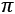radians (180°). Conversely, two points whose angle is very small can always be considered similar.

In this common case, we assume that the transition between concepts is semantically smooth, so two points belonging to different sets can always be compared according to their common features (for example, the boundary between warm and cold can be a point whose temperature is the average between the two groups). The only important thing to know is that if we move along the circle far from a point, increasing the angle, the dissimilarity increases. For our purposes, let's consider the points (-4, 0) and (-1, 3), which are almost orthogonal in the original distribution:

``````X_test = [
[-4., 0.],
[-1., 3.]
]
Y_test = nz.transform(X_test)
print(np.arccos(np.dot(Y_test, Y_test)))
``````

The output of the previous snippet is:

``````1.2490457723982544
``````

The dot product between two vectors x1 and x2 is equal to:

The last step derives from the fact that both vectors have unit norms. Therefore, the angle they form after the projection is almost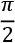, indicating that they are indeed orthogonal. If we multiply the vectors by a constant, their Euclidean distance will obviously change, but the angular distance after normalization remains the same. I invite you to check it!

Therefore, we can completely get rid of the relative Euclidean distances and work only with the angles, which, of course, must be correlated to an appropriate similarity measure.

### Whitening

Another very important preprocessing step is called whitening, which is the operation of imposing an identity covariance matrix to a zero-centered dataset:

As the covariance matrix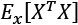is real and symmetrical, it's possible to eigendecompose it without the need to invert the eigenvector matrix:

The matrix V contains the eigenvectors as columns, and the diagonal matrix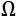contains the eigenvalues. To solve the problem, we need to find a matrix A, such that:

Using the eigendecomposition previously computed, we get:

Hence, the matrix A is:

One of the main advantages of whitening is the decorrelation of the dataset, which allows for an easier separation of the components. Furthermore, if X is whitened, any orthogonal transformation induced by the matrix P is also whitened:

Moreover, many algorithms that need to estimate parameters that are strictly related to the input covariance matrix can benefit from whitening, because it reduces the actual number of independent variables. In general, these algorithms work with matrices that become symmetrical after applying the whitening.

Another important advantage in the field of deep learning is that the gradients are often higher around the origin and decrease in those areas where the activation functions (for example, the hyperbolic tangent or the sigmoid) saturate (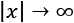). That's why the convergence is generally faster for whitened—and zero-centered—datasets.

In the following graph, it's possible to compare an original dataset and the result of whitening, which in this case is both zero-centered and with an identity covariance matrix:

Original dataset (left) and whitened version (right)

When a whitening process is needed, it's important to consider some important details. The first one is that there's a scale difference between the real sample covariance and the estimation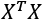, often adopted with the Singular Value Decomposition (SVD). The second one concerns some common classes implemented by many frameworks, such as scikit-learn's `StandardScaler`. In fact, while zero-centering is a feature-wise operation, a whitening filter needs to be computed considering the whole covariance matrix; `StandardScaler` implements only unit variance and feature-wise scaling.

Luckily, all scikit-learn algorithms that can benefit from a whitening preprocessing step provide a built-in feature, so no further actions are normally required. However, for all readers who want to implement some algorithms directly, I've written two Python functions that can be used for both zero-centering and whitening. They assume a matrix X with a shape (NSamples × n). In addition, the `whiten()` function accepts the parameter `correct`, which allows us to apply the scaling correction. The default value for `correct` is `True`:

``````import numpy as np
def zero_center(X):
return X - np.mean(X, axis=0)
def whiten(X, correct=True):
Xc = zero_center(X)
_, L, V = np.linalg.svd(Xc)
W = np.dot(V.T, np.diag(1.0 / L))
return np.dot(Xc, W) * np.sqrt(X.shape) if correct else 1.0
``````

### Training, validation, and test sets

As we have previously discussed, the numerosity of the sample available for a project is always limited. Therefore, it's usually necessary to split the initial set X, together with Y, each of them containing N i.i.d. elements sampled from pdata, into two or three subsets as follows:

• Training set used to train the model
• Validation set used to assess the score of the model without any bias, with samples never seen before
• Test set used to perform the final validation before moving to production

The hierarchical structure of the splitting process is shown in the following figure:

Hierarchical structure of the process employed to create training, validation, and test sets

Considering the previous diagram, generally, we have:

The sample is a subset of the potential complete population, which is partially inaccessible. Because of that, we need to limit our analysis to a sample containing N elements. The training set and the validation/test set are disjoint (that is, the evaluation is carried out using samples never seen during the training phase).

The test set is normally obtained by removing Ntest samples from the initial validation set and keeping them apart until the final evaluation. This process is quite straightforward:

1. The model M is trained using the training set
2. M is evaluated using the validation set and a designated Score(•) function
3. If Score(M) > Desired accuracy:

perform the final test to confirm the results

4. Otherwise, the hyperparameters are modified and the process restarts

Since the model is always evaluated on samples that were not employed in the training process, the Score(•) function can determine the quality of the generalization ability developed by the model. Conversely, an evaluation performed using the training sample can help us understand whether the model is basically able to learn the structure of the dataset. We'll discuss these concepts further over the next few sections.

The choice of using two (training and validation) or three (training, validation, and test) sets is normally related to the specific context. In many cases, a single validation set, which is often called the test set, is used throughout the whole process. That's usually because the final goal is to have a reliable set of i.i.d. elements that will never be employed for training and, consequently, whose prediction results reflect the unbiased accuracy of the model. In this book, we'll always adopt this strategy, using the expression test set instead of validation set.

Depending on the nature of the problem, it's possible to choose a split percentage ratio of 70% – 30%, which is a good practice in machine learning, where the datasets are relatively small, or a higher training percentage of 80%, 90%, or up to 99% for deep learning tasks where the numerosity of the samples is very high. In both cases, we're assuming that the training set contains all the information we'll require for a consistent generalization.

In many simple cases, this is true and can be easily verified; but with more complex datasets, the problem becomes harder. Even if we draw all the samples from the same distribution, it can happen that a randomly selected test set contains features that are not present in other training samples. When this happens, it can have a very negative impact on global accuracy and, without other methods, it can also be very difficult to identify.

This is one of the reasons why, in deep learning, training sets are huge: considering the complexity of the features and structure of the data generating the distributions, choosing large test sets can limit the possibility of learning particular associations. This is a consequence of an effect called overfitting, which we'll discuss later in this chapter.

In scikit-learn, it's possible to split the original dataset using the `train_test_split()` function, which allows specifying the train/test size, and if we expect to have randomly shuffled sets (which is the default). For example, if we want to split `X` and `Y`, with 70% training and 30% test, we can use:

``````from sklearn.model_selection import train_test_split
X_train, X_test, Y_train, Y_test = train_test_split(X, Y, train_size=0.7, random_state=1000)
``````

Shuffling the sets is always good practice, in order to reduce the correlation between samples (the method train_test_split has a parameter called shuffle that allows this to be done automatically). In fact, we have assumed that `X` is made up of i.i.d samples, but often two subsequent samples have a strong correlation, which reduces the training performance. In some cases, it's also useful to re-shuffle the training set after each training epoch; however, in the majority of our examples, we'll work with the same shuffled dataset throughout the whole process.

Shuffling has to be avoided when working with sequences and models with memory. In all those cases, we need to exploit the existing correlation to determine how the future samples are distributed. Whenever an additional test set is needed, it's always possible to reuse the same function: splitting the original test set into a larger component, which becomes the actual validation set, and a smaller one, the new test set that will be employed for the final performance check.

When working with NumPy and scikit-learn, it's always a good practice to set the random seed to a constant value, so as to allow other people to reproduce the experiment with the same initial conditions. This can be achieved by calling `np.random.seed(...)` and using the `random-state` parameter present in many scikit-learn methods.

### Cross-validation

A valid method to detect the problem of wrongly selected test sets is provided by the cross-validation (CV) technique. In particular, we're going to use the K-Fold cross-validation approach. The idea is to split the whole dataset X into a moving test set and a training set made up of the remaining part. The size of the test set is determined by the number of folds, so that during k iterations, the test set covers the whole original dataset.

In the following diagram, we see a schematic representation of the process:

K-Fold cross-validation schema

In this way, we can assess the accuracy of the model using different sampling splits, and the training process can be performed on larger datasets; in particular, on (k-1)N samples. In an ideal scenario, the accuracy should be very similar in all iterations; but in most real cases, the accuracy is quite below average.

This means that the training set has been built excluding samples that contain all the necessary examples to let the model fit the separating hypersurface considering the real pdata. We're going to discuss these problems later in this chapter. However, if the standard deviation of the accuracies is too large—a threshold must be set according to the nature of the problem/model—that probably means that X hasn't been drawn uniformly from pdata, and it's useful to evaluate the impact of the outliers in a preprocessing stage. In the following graph, we see the plot of 15-fold CV performed on a Logistic Regression:

Cross-validation accuracies

The values oscillate from 0.84 to 0.95, with an average of 0.91, marked on the graph as a solid horizontal line. In this particular case, considering the initial purpose was to use a linear classifier, we can say that all folds yield high accuracies, confirming that the dataset is linearly separable; however, there are some samples, which were excluded in the ninth fold, that are necessary to achieve a minimum accuracy of about 0.88.

K-Fold cross-validation has different variants that can be employed to solve specific problems:

• Stratified K-Fold: A standard K-Fold approach splits the dataset without considering the probability distribution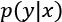, therefore some folds may theoretically contain only a limited number of labels. Stratified K-Fold, instead, tries to split X so that all the labels are equally represented.
• Leave-one-out (LOO): This approach is the most drastic because it creates N folds, each of them containing N-1 training samples and only one test sample. In this way, the maximum possible number of samples is used for training, and it's quite easy to detect whether the algorithm is able to learn with sufficient accuracy, or if it's better to adopt another strategy.
• The main drawback of this method is that N models must be trained, and when N is very large this can cause a performance issue. It's also an issue that with a large number of samples, the probability that two random values are similar increases, and therefore many of the folds will yield almost identical results. At the same time, LOO limits the possibilities for assessing the generalization ability of a model, because a single test sample is not enough for a reasonable estimation.
• Leave-P-out (LPO): In this case, the number of test samples is set to p non-disjoint sets, so the number of folds is equal to the binomial coefficient of n over p. This approach mitigates LOO's drawbacks, and it's a trade-off between K-Fold and LOO. The number of folds can be very high, but it's possible to control it by adjusting the number p of test samples; however, if p isn't small or big enough, the binomial coefficient can exponentially explode, as shown in the following figure in case of n=20 and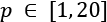:

Exploding effect of the binomial coefficient when p is about half of n

Scikit-learn implements all those methods, with some other variations, but I suggest always using the `cross_val_score()` function, which is a helper that allows applying the different methods to a specific problem. It uses Stratified K-Fold for categorical classifications and Standard K-Fold for all other cases. Let's now try to determine the optimal number of folds, given a dataset containing 500 points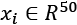with redundancies, internal non-linearities, and belonging to 5 classes:

``````from sklearn.datasets import make_classification
from sklearn.preprocessing import StandardScaler
X, Y = make_classification(n_samples=500, n_classes=5,
n_features=50, n_informative=10,
n_redundant=5, n_clusters_per_class=3,
random_state=1000)
ss = StandardScaler()
X = ss.fit_transform(X)
``````

As the first exploratory step, let's plot the learning curve using a Stratified K-Fold with 10 splits; this assures us that we'll have a uniform class distribution in every fold:

``````import numpy as np
from sklearn.linear_model import LogisticRegression
from sklearn.model_selection import learning_curve, StratifiedKFold
lr = LogisticRegression(solver='lbfgs', random_state=1000)
splits = StratifiedKFold(n_splits=10, shuffle=True, random_state=1000)
train_sizes = np.linspace(0.1, 1.0, 20)
lr_train_sizes, lr_train_scores, lr_test_scores = \
learning_curve(lr, X, Y, cv=splits, train_sizes=train_sizes,
n_jobs=-1, scoring='accuracy',
shuffle=True, random_state=1000)
``````

The result is shown in the following diagram:

Learning curves for a Logistic Regression classification

The training curve decays when the training set size reaches its maximum, and converges to a value slightly larger than 0.6. This behavior indicates that the model is unable to fully capture the dynamics of `X`, and it has good performances only when the training set size is very small (that is, the actual data generating process is not fully covered). Conversely, the test performances improve when the training set is larger. This is an obvious consequence of the wider experience that the classifier gains when more and more points are employed.

Considering both the training and test accuracy trends, we can conclude that in this case a training set larger than about 270 points doesn't yield any strong benefit. On the other hand, since the test accuracy is extremely important, it's preferable to use the maximum number of points. As we're going to discuss later in this chapter, it indicates how well the model generalizes. In this case, the average training accuracy is worse, but there's a small benefit in the test accuracy. I've chosen this example because it's a particular case that requires a trade-off. In many cases, the curves grow proportionally, and determining the optimal number of folds is straightforward.

However, when the problem is harder, as it is in this case—considering the nature of the classifier—the choice is not obvious, and analyzing the learning curve becomes an indispensable step. Before we move on, we can try to summarize the rule. We need to find the optimal number of folds so that cross-validation guarantees an unbiased measure of the performances.

As a dataset X is drawn from an underlying data generating process, the amount of information that X carries is bounded by pdata. This means that an increase of the dataset's size over a certain threshold can only introduce redundancies, which cannot improve the performance of the model. The optimal number of folds, or the size of the folds, can be determined by considering the point at which both training and test average accuracies stabilize. The corresponding training set size allows us to use the largest possible test sample size for performance evaluations. Let's now compute the average CV accuracies for a different number of folds:

``````import numpy as np
from sklearn.linear_model import LogisticRegression
from sklearn.model_selection import cross_val_score
mean_scores = []
cvs = [x for x in range(5, 100, 10)]
for cv in cvs:
score = cross_val_score(LogisticRegression(solver='lbfgs',
random_state=1000),
X, Y, scoring='accuracy', n_jobs=-1,
cv=cv)
mean_scores.append(np.mean(score))
``````

The result is shown in the following figure:

Average cross-validation accuracy for a different number of folds

The curve has a peak corresponding to 15-fold CV, which corresponds to a training set size of 466 points. In our previous analysis, we have discovered that such a value is close to the optimal one. On the other side, a larger number of folds implies smaller test sets.

We have seen that the average CV accuracy depends on a trade-off between training and test set sizes. Therefore, when the number of folds increases, we should expect an improvement in the performances. This result becomes clear with 85 folds. In this case, only 6 samples are used for testing purposes (1.2%), which means the validation is not particularly reliable, and the average value is associated with a very large variance (that is, in some lucky cases, the CV accuracy can be large, while in the remaining ones, it can be close to 0).

Considering all the factors, the best choice remains k=15, which implies the usage of 34 test samples (6.8%). I hope it's clear the right choice of k is a problem itself; however, in practice, a value in the range [5, 15] is often the most reasonable default choice. The goal of a good choice is also to maximize the stochasticity of CV and, consequently, to reduce the cross-correlations between estimations. Very small folds imply that many models are highly correlated, while over-large folds reduce the learning ability of the model. Therefore, a good trade-off should never prefer either very small values (acceptable only if the dataset is extremely small) nor over-large ones.

Of course, this value is strictly correlated to the nature of the task and to the structure of the dataset. In some cases, just 3 to 5% of test points can be enough to perform a correct assessment; in many other ones, a larger set is needed in order to capture the dynamics of all regions.

As a general rule, I always encourage the employment of CV for performance measurements. The main drawback of this method is its computational complexity. In the context of deep learning, for example, a training process can require hours or days, and repeating it without any modification of the hyperparameters can be unacceptable. In all these cases, a standard training-test set decomposition will be used, assuming that for both sets the numerosity is large enough to guarantee full coverage of the underlying data generating process.

# Characteristics of a machine learning model

In this section, we're mainly going to consider supervised models, even though the concepts we'll discuss are valid in general. We'll try to determine how it's possible to measure the theoretical potential accuracy of a model, and a model's ability to generalize correctly over every possible sample drawn from pdata.

The majority of these concepts were developed long before the deep learning age, but continue to have an enormous influence on research projects.

The idea of capacity, for example, is an open-ended question that neuroscientists keep on asking themselves about the human brain. Modern deep learning models with dozens of layers and millions of parameters have reopened this theoretical question from a mathematical viewpoint. Together with this, other elements, such as the limits for the variance of an estimator, have again attracted the limelight because the algorithms are becoming more and more powerful, and performances that once were considered far from feasible are now a reality.

Being able to train a model, so as to exploit its full capacity, maximize its generalization ability, and increase its accuracy to overcome even human performances, is what a modern data scientist or deep learning engineer ought to expect from their work.

## Learnability

Before starting the discussion of the features of a model, it's helpful to introduce some fundamental elements related to the concept of learnability, work not too dissimilar from the mathematical definition of generic computable functions. The first formal work on this was published by Valiant (in Valiant L., A theory of the learnable, Communications of the ACM, 27, 1984) and is mostly an introduction of the concept of Probably Approximately Correct (PAC) learning. We won't discuss the very technical mathematical details of PAC learning in this book, but it's useful to understand the possibility of finding a suitable way to describe a learning process, without referring to specific models.

For simplicity, let's assume we have a selector algorithm that can search a hypothesis hi in a set H. This element can be interpreted in many ways according to the context. For example, the set of hypotheses might correspond to the set of reasonable parameters of a model, or, in another scenario, to a finite set of algorithms tuned to solve specific problems. As the definition is general, we don't have to worry about its structure.

On the other side of this landscape, there is the set of concepts C that we want to learn. A concept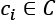is an instance of a problem belonging to a defined class. Again, the structure can vary, but for simplicity the reader can assume that a concept is associated with a classical training set containing a finite number of data points.

For our purposes, it's necessary to define the structure of an error measure (we're going to do that later, when talking about cost and loss functions). If you're not familiar with the concept, it's possible to consider the normalized average number of misclassified data points.

If the sample size is N, an error equal to 0 implies that there are no misclassifications, while an error equal to 1 means that all the samples have been misclassified. Just as for AUC diagrams, in a binary classifier we consider the threshold of 0.5 as lower bound, because it corresponds to a random choice of the label.

An informal definition states that a problem is PAC learnable if, given a desired maximum error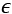and probability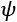, it's possible to set a minimum sample size N for every concept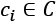, so that a selector algorithm can find a hypothesis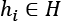such that the probability that the error is upper bounded byis greater than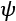.

As the problem is generally stochastic, the result must be expressed using probabilities. That means we can summarize the previous definition, saying that for a PAC learnable problem,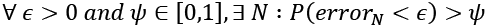. We also need to add that we expect the sample to have polynomial growth as a function of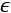and. This condition can be relaxed with respect to the original one, but it's enough to understand that a problem that requires an infinite sample size to achieve an error greater than 0 is not PAC learnable.

This characterization justifies the use of the word approximately in the definition, which could lead to misunderstandings if not fully mathematically defined. In our context, in fact, we cope with deterministic problems very rarely, and they generally don't require a machine learning algorithm. It's more helpful to know that the probability of obtaining a small error is always larger than a predefined threshold. Even if the theory is much more complex and rigorous, we can avoid all the theoretical details (which are available in Valiant's paper mentioned before) and limit our analysis to this concrete meaning of the concept.

Given a problem, we can generally find a model that can learn the associated concept and keep the accuracy above a minimum acceptable value. That's equivalent to saying that the concept is PAC learnable, a condition that we haven't proved, but that can reasonably be assumed to be true in most real-life contexts. Therefore, taking the PAC learnability for granted, we know that we can reach the desired accuracy for a specific scenario.

However, the price to pay for that isn't so easy to evaluate. For sure, when the requirements become stronger and stronger, we also need a larger training set and a more powerful model, but is this enough to achieve an optimal result? Moreover, is it possible to quantify how optimal the result is using a single measure? In the next sections, we'll introduce the elements that must be evaluated when defining, or evaluating, every machine learning model.

## Capacity of a model

If we consider a supervised model as a set of parameterized functions, we can define the representational capacity as the intrinsic ability of a certain generic function to map a relatively large number of data distributions. To understand this concept, let's consider a function f(x) that admits infinite derivatives, and rewrite it as a Taylor expansion around a starting point x0:

We can decide to take only the first n terms, so to have an n-degree polynomial function around the starting point x0 = 0:

Consider a simple bi-dimensional scenario with six functions, starting from a linear one. We can observe the different behaviors with a small set of data points:

Different behavior produced by six polynomial separating curves

The ability to rapidly change the curvature is proportional to the degree. If we choose a linear classifier, we can only modify its slope—the example is always in a bi-dimensional space—and the intercept.

Instead, if we pick a higher-degree function, we have more possibilities to bend the curvature when it's necessary. If we consider n=1 and n=2 in the plot (on the top-right, they are the first and the second functions), with n=2, we can include the dot corresponding to x=11, but this choice has a negative impact on the dot at x=5.

Only a parameterized non-linear function can solve this problem efficiently. That's because this simple problem requires a representational capacity higher than the one provided by linear classifiers. Another classical example is the XOR function. For a long time, several researchers opposed perceptrons (linear neural networks) because they couldn't classify a dataset generated by the XOR function.

Fortunately, the introduction of Multilayer Perceptrons (MLP), with non-linear functions, allowed us to overcome this problem, and many other problems whose complexity is beyond the possibilities of any classic machine learning model. For a better understanding of this concept, it's helpful to introduce a formalism that allows us to understand how different model families treat the same kind of problem, achieving better or worse accuracies.

### Vapnik-Chervonenkis capacity

A common mathematical formalization of the capacity of a classifier is provided by the Vapnik-Chervonenkis theory. To introduce the definition, it's first necessary to define the concept of shattering. If we have a class of sets C and a set M, we say that C shatters M if:

In other words, given any subset of M, it can be obtained as the intersection of a particular instance of C (cj) and M itself. Now, if we consider a model as a parameterized function:

Considering the variability of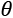, C can be considered as a set of functions with the same structure, but different parameters:

We want to determine the capacity of this model family in relation to a finite dataset X:

According to the Vapnik-Chervonenkis theory, we can say that the model family C shatters X if there are no classification errors for every possible label assignment. Therefore, we can define the Vapnik-Chervonenkis-capacity or VC-capacity—sometimes called VC-dimension—as the maximum cardinality of a subset of X, so that any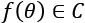can shatter it (that is, the maximum number of points that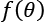can shatter).

For example, if we consider a linear classifier in a bi-dimensional space, the VC-capacity is equal to 3, because it's always possible to label three samples so that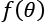shatters them. However, it's impossible to do it in all situations where N > 3. The XOR problem is an example that needs a VC-capacity higher than three. Let's explore the following plot:

XOR problem with different separating curves

This particular label choice makes the set non-linearly separable. The only way to overcome this problem is to use higher-order functions, or non-linear ones. The curve lines—belonging to a classifier whose VC-capacity is greater than 3—can separate both the upper-left and the lower-right regions from the remaining space, but no straight line can do the same, although it can always separate one point from the other three.

This definition of capacity is quite rigorous (the reader who's interested in the all theoretical aspects can read Mohri M., Rostamizadeh A., Talwalkar A., Foundations of Machine Learning, Second edition, The MIT Press, 2018), but it can help understand the relation between the complexity of a dataset and a suitable model family. According to the principle of Occam's razor, the simplest model that obtains an optimal accuracy (that is, the optimal set of measures that quantifies the performances of an algorithm) must be selected, and in this book, we are going to repeat this principle many times. However, the reason for this advice, which is strictly connected to the informal definition of PAC learning, will become obvious after having introduced the concepts of bias and variance of an estimator.

## Bias of an estimator

Let's now consider a parameterized model with a single vectoral parameter. This isn't a limitation, just a didactic choice:

The goal of a learning process is to estimate the parameter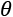so as, for example, to maximize the accuracy of its classifications. We define the bias of an estimator in relation to a parameter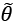:

In other words, the bias of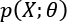is the difference between the expected value of the estimation and the real parameter value. Remember that the estimation is a function of X, and cannot be considered a constant in the sum.

An estimator is said to be unbiased if:

Moreover, the estimator is defined as consistent if the sequence of estimations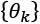ofconverges in probability to the real value when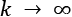(that is, it is asymptotically unbiased):

It's obvious that this definition is weaker than the previous one, because in this case, we're only certain of achieving unbiasedness if the sample size becomes infinitely large. However, in practice, many asymptotically unbiased estimators can be considered unbiased when k > Nk. In other words, with samples containing at least Nk points, the results have a negligible error, and the estimation can be considered correct. From the theory, we know that some model families are unbiased (for example, linear regressions optimized using the ordinary least square), but confirming a model is unbiased is extremely different to test when the model is very complex.

For example, we can suppose that a deep neural network is prone to be unbiased, but as we are going to discuss throughout the book, the sample size is a fundamental parameter in achieving good results. Given a dataset X whose samples are drawn from pdata, the accuracy of an estimator is inversely proportional to its bias. Low-bias (or unbiased) estimators are able to map the dataset X with high-precision levels, while high-bias estimators are very likely to have too low a capacity for the problem to solve, and therefore their ability to detect the whole dynamic is poor.

This also implies that, in many cases, if k << Nk, the sample doesn't contain enough of the representative elements that are necessary to rebuild the data generating process, and the estimation of the parameters risks becoming clearly biased. Remember that the training set X is drawn from pdata and contains a limited number of points. Hence, given k different sets X1, X2, ..., Xk obtained from the same data generating process, we are interested in understanding whether the initial estimation is still valid.

If X is truly representative of pdata and the estimator is unbiased, we should expect to always obtain the same mean, with a reasonable tolerance. This condition assures that, at least on average, the estimator yields results distributed around the true values. Let's now consider the extremes of this process: underfitting and overfitting a model.

### Underfitting

A model with a large bias is likely to underfit the training set X (that is, it's not able to learn the whole structure of X). Let's consider the simple bidimensional scenario shown in the following figure:

Underfitted classifier: The curve cannot separate correctly the two classes

Even if the problem is very hard, we could try to adopt a linear model and, at the end of the training process, the slope and the intercept of the separating line are about 1 and -1, as shown in the plot. However, if we measure the accuracy, we discover that it's not as large as expected—indeed, it's about 0.65—because there are too many class 2 samples in the region assigned to class 1.

Moreover, the sample size of 200 points is quite small and, therefore, X cannot be a true representative of the underlying data generating process. Considering the density for class 2 observed in the area x0 < 0 and 1 < x1 < 2, it's reasonable to suppose that larger sample size could lead to a worse accuracy due to the increased number of misclassified class 2 points. Independent of the number of iterations, this model will never be able to learn a good association between X and Y.

This condition is called underfitting, and the major indicator of underfitting is very low training accuracy. Unfortunately, even if some data preprocessing steps can improve the accuracy, when a model is underfitted, the only valid solution is to adopt a higher-capacity model. In fact, when the estimation of the parameters is biased, its expected value is always different from the true value. That difference leads to a systematic prediction error that cannot be corrected.

Considering the previous example, a linear model (for example, a logistic regression) can only modify the slope and the intercept of the separating line. The reader can easily see that the number of degrees of freedom are too small to achieve, for example, an accuracy greater than 0.95. Instead, using a polynomial classifier (for example, a parabolic one), the problem can be easily solved. The introduction of another parameter. the coefficient of the square term, allows defining a curve separating line that surely leads to a better fit. Of course, the price to pay is double:

• A model with larger capacity needs a higher computational effort. This is often a secondary problem.
• The extra capacity could reduce the generalization ability, if X is not fully representative of pdata (we are going to discuss this problem in the next section).

In a machine learning task, our goal is to achieve the maximum accuracy, starting from the training set and then moving on to the validation set. More formally, we can say that we want to improve our models so to get as close as possible to Bayes error, which is the theoretical minimal generalization error achievable by an estimator. It can also be expressed as Bayes accuracy, which is the maximum achievable generalization accuracy.

In the following diagram, we can see a representation of this process:

Accuracy level diagram

Bayes accuracy is often a purely theoretical limit and, for many tasks, it's almost impossible to achieve, even using biological systems. However, advancements in the field of deep learning allow creating models that have a target accuracy slightly below the Bayes one. In general, there's no closed form for determining the Bayes accuracy, therefore human abilities are considered as a benchmark.

In the previous classification example, a human being is immediately able to distinguish among different dot classes, but the problem can be very hard for a limited-capacity classifier. Some of the models we're going to discuss can solve this problem with a very high target accuracy, but at this point, we need to introduce  the concept of variance of an estimator in order to understand the effects of excessive capacity.

## Variance of an estimator

At the beginning of this chapter, we defined the data generating process pdata, and we've assumed that our dataset X has been drawn from this distribution. However, we don't want to learn existing relationships limited to X; we expect our model to be able to generalize correctly to any other subset drawn from pdata. A good measure of this ability is provided by the variance of the estimator:

The variance can be also defined as the square of the standard error, analogously to the standard deviation. A large variance implies dramatic changes in accuracy when new subsets are selected. In fact, even if the model is unbiased, and the estimated values of the parameters are spread around the true mean, they can show high variability.

For example, suppose that an estimated parameter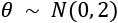and the true mean is actually 0. We know that the probability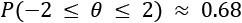; hence, if a wrong estimation that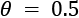can lead to a significant error, there's a very high risk of misclassification with the majority of validation samples. This effect is related to the fact that the model has probably reached a very high training accuracy through over-learning a limited set of relationships, and it has almost completely lost its ability to generalize (that is, the average validation accuracy decays when never-seen samples are tested).

However, if it's possible to obtain unbiased estimators, it's almost impossible to reduce the variance under a well-defined threshold (see the later section related to the Cramér-Rao bound). Before discussing the implications of the variance, we need to introduce the opposite extreme situation to underfitting: overfitting a model.

### Overfitting

If underfitting was the consequence of low capacity and large bias, overfitting is a phenomenon strictly related to large variance. In general, we can observe a very high training accuracy (even close to the Bayes level), but not a poor validation accuracy.

This means that the capacity of the model is high enough or even excessive for the task (the higher the capacity, the higher the probability of large variances), and that the training set isn't a good representation of pdata. To understand the problem, consider the following classification scenarios:

Acceptable fitting (left), overfitted classifier (right)

The left plot has been obtained using logistic regression, while the right plot was obtained with an SVM algorithm with a sixth-degree polynomial kernel. If we consider the second model, the decision boundaries seem much more precise, with some samples just over them. Considering the shapes of the two subsets, it would be possible to say that a non-linear SVM can better capture the dynamics; however, if we sample another dataset from pdata and the diagonal tail becomes wider, logistic regression continues to classify the points correctly, while the SVM accuracy decreases dramatically.

The second model is very likely to be overfitted, and some corrections are necessary. When the validation accuracy is much lower than the training one, a good strategy is to increase the number of training samples, to consider the real pdata. In fact, it can happen that a training set is built starting from a hypothetical distribution that doesn't reflect the real one, or the number of samples used for the validation is too high, reducing the amount of information carried by the remaining samples.

Cross-validation is a good way to assess the quality of datasets, but it can always happen that we misclassify completely new subsets (for example, generated when the application is deployed in a production environment), even if they were supposed to belong to pdata. If it's not possible to enlarge the training set, data augmentation could be a valid solution, because it allows creating artificial samples (for images, it's possible to mirror, rotate, or blur them) starting from the information stored in the known ones.

Other strategies to prevent overfitting are based on a technique called regularization, which we're going to discuss in the next chapter. For now, we can say that the effect of regularization is similar to a partial linearization, which implies a capacity reduction with a consequent variance decrease and a tolerable bias increase.

### The Cramér-Rao bound

If it's theoretically possible to create an unbiased model, even asymptotically, this is not true for the variance. To understand this concept, it's necessary to introduce an important definition: the Fisher information. If we have a parameterized model and a data-generating process pdata, we can define a likelihood function by considering the following parameters:

This function allows us to measure how well the model describes the original data generating process. The shape of the likelihood can vary substantially, from well-defined, peaked curves, to almost flat surfaces. Let's consider the following graph, showing two examples based on a single parameter. The x-axis represents the value of a generic parameter, while the y-axis is the log-likelihood:

Very peaked likelihood (left), flatter likelihood (right)

We can immediately understand that, in the first case, the maximum likelihood (which represents the value for which the model has the highest probability to generate the training dataset – the concept will be discussed in a dedicated section) can be easily reached using classic optimization methods, because the surface is very peaked. In the second case, instead, the gradient magnitude is smaller, and it's rather easy to stop before reaching the actual maximum because of numerical imprecisions or tolerances. In worst cases, the surface can be almost flat in very large regions, with a corresponding gradient close to zero.

Of course, we'd like it if we could always work with very sharp and peaked likelihood functions, because they carry more information about their maximum. More formally, the Fisher information quantifies this value. For a single parameter, it is defined as follows:

The Fisher information is an unbounded, non-negative number, that is proportional to the amount of information carried by the log-likelihood; the use of logarithm has no impact on the gradient ascent, but it simplifies complex expressions by turning products into sums.

This value can be interpreted as the speed of the gradient when the function is reaching the maximum; therefore, higher values imply better approximations, while a hypothetical value of zero means that the probability to determine the right parameter estimation is also null.

When working with a set of K parameters, the Fisher information becomes a positive semidefinite matrix:

This matrix is symmetrical, and also has another important property: when a value is zero, it means that the corresponding parameters are orthogonal for the purpose of the maximum likelihood estimation, and they can be considered separately. In many real cases, if a value is close to zero, it determines a very low correlation between parameters. In that case, even if it's not mathematically rigorous, it's possible to decouple them anyway.

At this point, we can introduce the Cramér-Rao bound, which states that for every unbiased estimator that adopts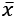with probability distribution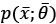as a measure set, the variance of any estimator of a parameter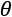is always lower-bounded according to the following inequality:

In fact, if we initially consider a generic estimator, and exploit Cauchy-Schwarz inequality with the variance and the Fisher information, which are both expressed as expected values, we obtain:

Now, if we need to compute the expression for derivatives of the bias with respect to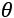:

Considering that the expected value of the estimation of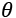doesn't depend on x, we can rewrite the right side of the inequality as:

If the estimator is unbiased, the derivative on the right side is equal to zero, and therefore, we get:

In other words, we can try to reduce the variance, but it will be always lower-bounded by the inverse Fisher information. Therefore, given a dataset and a model, there's always a limit to the ability to generalize.

In some cases, this measure is easy to determine; however, its real value is theoretical, because it provides the likelihood function with another fundamental property: it carries all the information needed to estimate the worst case for the variance. This is not surprising: when we discussed the capacity of a model, we saw how different functions could lead to higher or lower accuracies. If the training accuracy is high enough, this means that the capacity is appropriate or even excessive for the problem; however, we haven't considered the role of the likelihood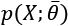.

Large-capacity models, in particular, with small or low-informative datasets, can lead to flat likelihood surfaces with a higher probability than lower-capacity models. Therefore, the Fisher information tends to become smaller, because there are more and more parameter sets that yield similar probabilities; this, at the end of the day, leads to higher variances and an increased risk of overfitting.

At this point, it's possible to fully understand the meaning of the empirical rule derived from the Occam's razor principle: if a simpler model can explain a phenomenon with enough accuracy, it doesn't make sense to increase its capacity.

A simpler model is always preferable when the performance is good and it represents the specific problem accurately, because it's normally faster and more efficient in both the training and the inference phases. When we talk about deep neural networks, this principle can be applied in a more precise way, because it's easier to increase or decrease the number of layers and neurons until the desired accuracy has been achieved.

# Summary

In this chapter, we discussed some fundamental concepts shared by almost any machine learning model.

In the first part, we introduced the data generating process, as a generalization of a finite dataset, and discussed the structure and properties of a good dataset. We discussed some common preprocessing strategies and their properties, such as scaling, normalizing, and whitening. We explained the most common strategies to split a finite dataset into a training block and a validation set, and we introduced cross-validation, with some of the most important variants, as one of the best approaches to avoid the limitations of a static split.

In the second part, we discussed the features of a machine learning model, and the concept of learnability. We discussed the main properties of an estimator: capacity, bias, and variance. We also introduced the Vapnik-Chervonenkis theory, which is a mathematical formalization of the concept of representational capacity, and we analyzed the effects of high biases and high variances. In particular, we discussed effects called underfitting and overfitting, defining the relationship with high bias and high variance.

In the next chapter, Chapter 2, Loss functions and Regularization , we're going to introduce loss and cost functions, which provide a simple and effective tool to fit machine learning models by minimizing an error measure or maximizing a specific objective.

• Darwiche A., Human-Level Intelligence or Animal-Like Abilities?, Communications of the ACM, Vol. 61, 10/2018
• Crammer K., Kearns M., Wortman J., Learning from Multiple Sources, Journal of Machine Learning Research, 9/2008
• Mohri M., Rostamizadeh A., Talwalkar A., Foundations of Machine Learning, Second edition, The MIT Press, 2018
• Valiant L., A theory of the learnable, Communications of the ACM, 27, 1984
• Ng A. Y., Feature selection, L1 vs. L2 regularization, and rotational invariance, ICML, 2004
• Dube S., High Dimensional Spaces, Deep Learning and Adversarial Examples, arXiv:1801.00634 [cs.CV]
• Sra S., Nowozin S., Wright S. J. (edited by), Optimization for Machine Learning, The MIT Press, 2011
• Bonaccorso G., Machine Learning Algorithms, Second Edition, Packt, 2018